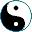We are in Set category. Take an set A, and let's define the following functor: X ↦ (X×A)A We can think of A as a machine's set of states; then (X × A)A consists of all state machines on X with output to X, that is, all functions A → (A × X), the first component being transition, and the second an output to X. Why is it a monad? uX : X → (A × X)A maps any element x ∊ X to a function that is identity on A and the constant x on X. Let me express it in Lisp: ```(define (ux x) (lambda (a) (list a x)))``` How about mX : (A × (A × X)A)A → (A × X)A? (this page continues on the next page)